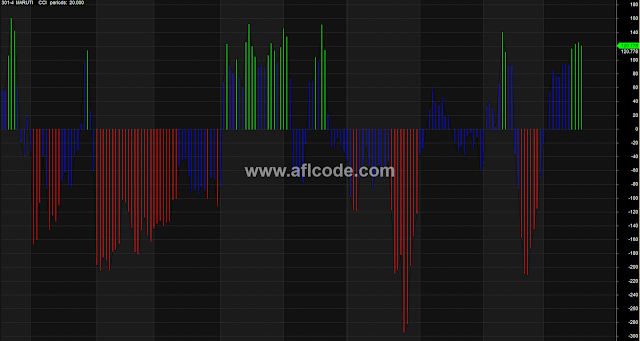### Colored CCI HistogramColored CCI Histogram

```//www.aflcode.com

T = 20;
Title = "301-4  " + Name () + "   "  + FullName() + "  "
+ "CCI" + "   " + "periods:" + WriteVal(T);
MaxGraph = 5;
Graph2 = CCI (T);

Uptrend = 100;  Downtrend =  -100;

Graph2BarColor = IIf (Graph2 > uptrend, 5,
IIf (Graph2 < downtrend,4,6));

Graph4 = Graph2;

Buy = Cross (Graph2, 0) AND (Ref (Graph2,-1) < 0) AND (Ref (Graph2,-2) < 0)AND (Ref (Graph2,-3) < 0) AND (LLV (RSI(9),10) < 30) AND Cross (RSI (9),50);
```

Previous
Next Post »